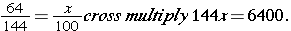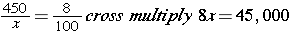# Percent Application

In this lesson, you will apply what you know about representing proportional relationships with a focus on solving problems with percents. Let us take a look at some types of percent problems we will be solving in this lesson.

Salespeople often work for commission. A commission is a fee paid to a person who makes a sale. It is usually a percent of the selling price. This percent is called the commission rate.

Sometimes, these salespeople are paid a commission plus a regular salary. The total pay is a percent of the sales they make plus a salary.Gratuity/Tip – is a percent added to the cost of the service (meal) and is also something that service employees depend on for much of their income.

Sales tax is the tax on the sale of an item or service. It is a percent of the purchase price and is collected by the seller.

Discount is a percent that is subtracted from the cost of an item. Often, there is a discount when an item is on sale.

Note! Any time you work with percents, there is room for error in calculations, so it is a good idea to estimate before you solve the problems. Then, you will have something valid with which to check your work.

### Example 1

Here is an example of how to use percents.

Rachel ate dinner with a group of friends. The restaurant would not give them separate checks, so each person had to calculate what she owed. Rachel’s entrée, drink, and dessert cost a total of \$9.75. If the sales tax rate is 8% and she wants to leave a 15% tip, how much should she pay?

Strategy – The answer is going to be the total amount dinner will cost Rachel. List the information necessary to solve.

• Food and drink totals \$9.75
• Sales tax is 8%
• Tip will be 15%

Estimate the percent that you will be adding to the total food bill of \$9.75.

Second, add the 15 and 8 together to get 23%.

There are two ways to approach this. You could multiply the cost by .23 and then add it to 9.75 or just multiply \$9.75 by 1.23 – (total amount plus the percents). This would give you \$11.9925, or about \$12.00.

How would you find the following?

21% of 66

66 x 0.21 = 13.86

A mental math approach:

20% is twice 10%, which is 6.6 + 6.6 = 13.2  1% is .66

20% + 1% = 13.2 + .66 = 13.86

36% of 120

120 x .36 = 43.2

A mental math approach:

10% + 10% + 10% + 5% + 1%

12 + 12 + 12 + 6 + 1.2 = 43.2

We know how to find the percent increase and percent decrease by using formulas in the form of proportions. You can also set up proportions to find the percent of a number.What percent of 144 is 64?The percent is 44.4%

We can take this same idea and turn it into a word problem. Emma earns 8% commission on her weekly sales. Last week, she earned \$450.00. How much merchandise did Emma sell last week? Restate the problem so you can set it up properly. \$450 is 8% of what? Now apply the formula:The totals sales are \$5625.00

Let’s look at percents as they apply to sales tax and discounts.

Marcy went shopping for a prom dress. She had \$170 to spend and was hoping to be able to purchase earrings in addition to a dress. The department store had the perfect dress for her. The dress was \$200, but was on sale at a discount of 25%. The sales tax in Marcy’s town was 7%. This is a multi-step problem so let us figure out the steps.

Find 25% of \$200 and subtract from the total amount of the dress.

Can you think of an easier way to find discount? If we are getting 25% off of the total price, we are paying 75% of the price, so just multiply by 75% and you do not have to subtract or anything.

.25 * 200 = 50 \$200-\$50 = 150

.75 * 200 = 150

Now, we know that the dress is going to cost \$150 before tax.

Normally, we would multiply the decimal form of the tax rate by the merchandise price and add that to get our total. But, since we know we have to pay the full \$150, we can multiply by one plus the tax to save time.

\$150 * 0.07 = 10.50

\$150 + 10.50 = \$160.50

1.07 * \$150 = \$160.50

The total cost of the dress is going to be \$160.50 after tax. It looks like she may be able to afford earrings after all.

Think about it! We learned earlier about percent increase and decrease. Markups, sales taxes, and discounts are really just different types of percent increases and decreases.

Take a look at the video to see how different strategies can be used for solving a percent application problems. Take notes and use the one(s) that works best for you!

Now see if you can use cross multiplying, combining, estimating, and other strategies to find the right solutions for the following problems.

## Percent Application Practice

1. PANTS \$ 80

Buy one at regular price. Get a second one for half price. (How much for 2 pairs?)

2. SWEATER \$50

Buy two. Get one free. How much for 3?

3. JACKET \$100

This item is marked down by 10%. Use a coupon and get 10% off the sale price. How much can you get it for?

4. Long Sleeved Tee- \$48

“50% off — Today Only Get Another 50% off”. How much can you get it for?

5. Find the total commission for Saul if his total sales are \$16,772 and the commission rate is 15%. A commission is what you are paid based on how much you sell.

6. Find the total monthly pay for Jane if total sales for a month were \$35,450, the commission rate is 4.5% (how much she is paid of the sales she makes), and the weekly base salary is \$175. Assume four weeks of work.

7. An item priced at \$776 has a sales tax of \$48.50. Find the sales tax rate expressed as a percent.

(source)